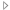## RF and Optics

Expand/Collapse all
AC/DC 模块

MEMS 模块

RF 模块

Select any check box to highlight individual products:
 Physics Interfaces and Study Types Electromagnetic Waves, Beam Envelopes Boundary Mode Analysis Eigenfrequency Frequency Domain Frequency-Domain Modal Wavelength Domain Electromagnetic Waves, Frequency Domain Boundary Mode Analysis Eigenfrequency Frequency Domain Frequency-Domain Modal Mode Analysis Wavelength Domain Electromagnetic Waves, Time Explicit Time Dependent Electromagnetic Waves, Transient Eigenfrequency Time Dependent Time-Dependent Modal Geometrical Optics Bidirectionally Coupled Ray Tracing Ray Tracing Transmission Line Eigenfrequency Frequency Domain Boundary Conditions Electromagnetic Waves, Beam Envelopes Electric Field Field Continuity Magnetic Field Matched Boundary Condition Perfect Electric Conductor Perfect Magnetic Conductor Periodic Condition Port Scattering Boundary Condition Surface Current Electromagnetic Waves, Frequency Domain Electric Field Lumped Element Lumped Port, Including Connection to Electrical Circuit Magnetic Field Perfect Electric Conductor Perfect Magnetic Conductor Periodic Condition Port Scattering Boundary Condition Surface Current Density Two-Port NetworkImpedance Boundary Condition Surface RoughnessTransition Boundary Condition Surface Current Density Surface Roughness Electromagnetic Waves, Time Explicit Electric Field Flux/Source Low-Reflecting Boundary Magnetic Field Perfect Electric Conductor Perfect Magnetic Conductor Surface Current Density Electromagnetic Waves, Transient Lumped Element Lumped Port, Including Connection to Electrical Circuit Magnetic Field Perfect Electric Conductor Perfect Magnetic Conductor Periodic Condition Scattering Boundary Condition Surface Current Geometrical Optics Accumulator Circular Wave Retarder Deposited Ray Power Grating with Diffraction Orders Ideal Depolarizer Linear Polarizer Linear Wave Retarder Material Discontinuity Mueller Matrix Ray Detector Thin Dielectric FilmWall Diffuse Scattering Disappear Freeze General Reflection Mixed Diffuse and Specular Reflection Pass Through Specular Reflection Stick Transmission Line Absorbing Boundary Incoming Wave Lumped Port Open Circuit Short Circuit Terminating Impedance Consitutive Relations Electromagnetic Waves, Beam Envelopes Anisotropic Material Conduction Linearized Resistivity Electric Displacement Debye Dispersion Dielectric Losses Drude-Lorentz Dispersion Loss Tangent Refractive Index Relative Permittivity Sellmeier Dispersion Magnetic Constitutive Relation Magnetic Losses Relative Permeability Electromagnetic Waves, Frequency Domain Anisotropic Material Porous Media and Mixture Materials Conduction Archie’s Law Linearized Resistivity Electric Displacement Debye Dispersion Dielectric Losses Drude-Lorentz Dispersion Loss Tangent Refractive Index Relative Permittivity Sellmeier Dispersion Magnetic Constitutive Relation Magnetic Losses Relative Permeability Electromagnetic Waves, Time Explicit Anisotropic Material Electromagnetic Waves, Transient Anisotropic Material Porous Media and Mixture Materials Conduction Archie’s Law Linearized Resistivity Electric Displacement Drude-Lorentz Dispersion Model Polarization Refractive Index Relative Permittivity Remanent Electric Displacement Magnetic Constitutive Relation Magnetization Relative Permeability Remanent Flux Density Lumped Parameters Touchstone File ExportS-Parameter Matrix Calculation S, Y and Z parameter conversions Pair, Edge, and Point Conditions Electromagnetic Waves, Beam Envelopes Continuity (pair) Electric Field (pair) Perfect Electric Conductor (pair) Perfect Magnetic Conductor (pair) Surface Current (pair) Electromagnetic Waves, Frequency Domain Continuity (pair) Edge Current (edge) Electric Field (pair) Electric Point Dipole (point) Magnetic Current (edge) Magnetic Point Dipole (point) Perfect Electric Conductor (pair) Perfect Magnetic Conductor (pair) Surface Current (pair) Electromagnetic Waves, Time Explicit Continuity (pair) Electromagnetic Waves, Transient Continuity (pair) Edge Current (edge) Electric Point Dipole (point) Magnetic Point Dipole (point) Perfect Electric Conductor (pair) Perfect Magnetic Conductor (pair) Surface Current (pair) Transmission Line Continuity (pair) Predefined Multiphysics InterfacesRay Heating Multiphysics Couplings Ray Heat Source Physics Interfaces Geometrical Optics Heat Transfer in Solids Ray Properties and Releases Geometrical Optics Auxiliary Dependent Variables Calculation of Ray Intensity and Polarization Corrections for Strongly Absorbing Media Illuminated Surface Inlet Nonlocal Accumulator Optical Path Length Calculation Phase Calculation Photometric Data Import Ray Detector Ray Properties Ray Termination Ray Tracing in Unmeshed Domains Release Release from Data File Release from Edge Release from Grid Release from Point Solar RadiationRay Release Distributions Conical Expression Hemispherical Lambertian Spherical Results and Visualization 2D Histogram Aberration Evaluation Far Field (plot) Interference Pattern Intersection Point 2D (data set) Intersection Point 3D (data set) Optical Aberration Phase Portrait Poincaré Map Ray (data set) Ray (plot) Ray Evaluation Ray TrajectoriesSmith (plot) Admittance Graph Impedance Graph Reflection Graph Volumetric Domain Properties 2D and Axisymmetric Electric Formulations In-Plane Vector Out-of-Plane Vector Three-Vector Electromagnetic Waves, Beam Envelopes Infinite Domain Modeling with Perfectly Matched Layer PolarizationWave Equation, Beam Envelopes Bidirectional Unidirectional Electromagnetic Waves, Frequency Domain External Current Density Infinite Domain Modeling with Perfectly Matched Layer PolarizationFar-Field Domain Far-Field and Directivity CalculationWave Equation, Electric Full Field Formulation Scattered Field Formulation Electromagnetic Waves, Time Explicit Electric Current Desity Infinite Domain Modeling with Absorbing Layer Magnetic Current Density Wave Equation, Electric and Magnetic Electromagnetic Waves, TransientWave Equation, Electric Drude-Lorentz Polarization Geometrical Optics Accumulator Deposited Ray Power Medium Properties Ray Heat Source Transmission Line Transmission Line Equation